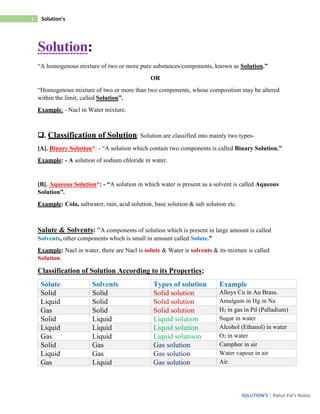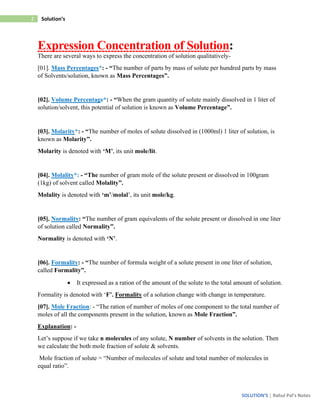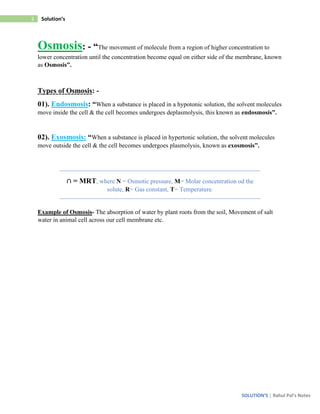Se está descargando tu SlideShare. ×

# Solution Chemistry NCERT Syllabus Notes 12th.pdf

Anuncio
Anuncio
Anuncio
Anuncio
Anuncio
Anuncio
Anuncio
Anuncio
Anuncio
Anuncio
AnuncioAnuncio
Cargando en…3
×

1 de 7 Anuncio

# Solution Chemistry NCERT Syllabus Notes 12th.pdf

n chemistry, a solution is a special type of homogeneous mixture composed of two or more substances. In such a mixture, a solute is a substance dissolved in another substance, known as a solvent.

n chemistry, a solution is a special type of homogeneous mixture composed of two or more substances. In such a mixture, a solute is a substance dissolved in another substance, known as a solvent.

Anuncio
Anuncio

### Solution Chemistry NCERT Syllabus Notes 12th.pdf

1. 1. 1 Solution’s SOLUTION’S | Rahul Pal’s Notes Solution: “A homogenous mixture of two or more pure substances/components, known as Solution.” OR “Homogenous mixture of two or more than two components, whose composition may be altered within the limit, called Solution”. Example: - Nacl in Water mixture. ❑. Classification of Solution: Solution are classified into mainly two types- [A]. Binary Solution*: - “A solution which contain two components is called Binary Solution.” Example: - A solution of sodium chloride in water. [B]. Aqueous Solution*: - “A solution in which water is present as a solvent is called Aqueous Solution”. Example: Cola, saltwater, rain, acid solution, base solution & salt solution etc. Salute & Solvents: “A components of solution which is present in large amount is called Solvents, other components which is small in amount called Solute.” Example: Nacl in water, there are Nacl is solute & Water is solvents & its mixture is called Solution. Classification of Solution According to its Properties; Solute Solvents Types of solution Example Solid Solid Solid solution Alloys Cu in Au Brass. Liquid Solid Solid solution Amalgum in Hg in Na Gas Solid Solid solution H2 in gas in Pd (Palladium) Solid Liquid Liquid solution Sugar in water Liquid Liquid Liquid solution Alcohol (Ethanol) in water Gas Liquid Liquid solutison O2 in water Solid Gas Gas solution Camphor in air Liquid Gas Gas solution Water vapour in air Gas Liquid Gas solution Air.
2. 2. 2 Solution’s SOLUTION’S | Rahul Pal’s Notes Expression Concentration of Solution: There are several ways to express the concentration of solution qualitatively- . Mass Percentages*: - “The number of parts by mass of solute per hundred parts by mass of Solvents/solution, known as Mass Percentages”. . Volume Percentage*: - “When the gram quantity of solute mainly dissolved in 1 liter of solution/solvent, this potential of solution is known as Volume Percentage”. . Molarity*: - “The number of moles of solute dissolved in (1000ml) 1 liter of solution, is known as Molarity”. Molarity is denoted with ‘M’, its unit mole/lit. . Molality*: - “The number of gram mole of the solute present or dissolved in 100gram (1kg) of solvent called Molality”. Molality is denoted with ‘m’/molal’, its unit mole/kg. . Normality: “The number of gram equivalents of the solute present or dissolved in one liter of solution called Normality”. Normality is denoted with ‘N’. . Formality: - “The number of formula weight of a solute present in one liter of solution, called Formality”. • It expressed as a ration of the amount of the solute to the total amount of solution. Formality is denoted with ‘F’. Formality of a solution change with change in temperature. . Mole Fraction: - “The ration of number of moles of one component to the total number of moles of all the components present in the solution, known as Mole Fraction”. Explanation: - Let’s suppose if we take n molecules of any solute, N number of solvents in the solution. Then we calculate the both mole fraction of solute & solvents. Mole fraction of solute = “Number of molecules of solute and total number of molecules in equal ratio”.
3. 3. 3 Solution’s SOLUTION’S | Rahul Pal’s Notes Osmosis: - “The movement of molecule from a region of higher concentration to lower concentration until the concentration become equal on either side of the membrane, known as Osmosis”. Types of Osmosis: - 01). Endosmosis: “When a substance is placed in a hypotonic solution, the solvent molecules move inside the cell & the cell becomes undergoes deplasmolysis, this known as endosmosis”. 02). Exosmosis: “When a substance is placed in hypertonic solution, the solvent molecules move outside the cell & the cell becomes undergoes plasmolysis, known as exosmosis”. ∩ = MRT, where N = Osmotic pressure, M= Molar concentration od the solute, R= Gas constant, T= Temperature. Example of Osmosis- The absorption of water by plant roots from the soil, Movement of salt water in animal cell across our cell membrane etc.
4. 4. 4 Solution’s SOLUTION’S | Rahul Pal’s Notes Solubility*: - “The maximum amount that can dissolved in specific amount of solvent under given set of condition”. Solubility depends on nature of solute & solvent as well as the condition of temperature & pressure. Saturated Solution: “A solution at which no more solute can be dissolved at a given condition of temperature & pressure is called Saturated Solution”. The amount of solute dissolved in a given amount of solvent is known as Solubility. ❑. Factor affecting the solubility of solid in liquid- Nature of solute, temperature. ❑. Factor affecting the solubility of gases- The nature of gas & the nature of solvent, effects of temperature, effect of pressure. Henry Law Constant: - “The solubility of a gas at a given temperature is directly proportional to the pressure at which it is dissolved”. If the mole fraction of gas xB at a given temperature as a measure of its solubility & P is the partial pressure of the gas in equilibrium with the solution then according to the Henry’s Law- xB<P or P<xB or P= KH.xB Where KH is constant called Henry Law Constant. Limitation of Henry Law: - a) The pressure of the gas is not too high. b) The temperature is not too high. c) The gas should not undergo any chemical reaction with the solvent. d) The has should not undergo dissociation in solution.
5. 5. 5 Solution’s SOLUTION’S | Rahul Pal’s Notes Vapour Pressure*: - “The pressure exerted by the vapour above the liquid surface in equilibrium with the liquid at a given temperature is called Vapour Pressure of the liquid”. It depends upon the above- 01. Nature of liquid- Liquid which have weak intermolecular forces are volatile & have greater vapour pressure. 02. Temperature- Vapour pressure increased with increase in temperature. Osmotic Pressure*: - “The Pressure which are mainly applied to the solution side which are commonly flowed on lower concentration to higher concentration, to stop those fluid movement, known as Osmotic pressure”. Or “The minimum pressure that stops the osmosis is equal to the osmotic pressure of the solution, called Osmotic pressure”. Osmotic pressure is denoted with ‘P’, The unit of osmotic pressure is Atomic pressure (ATM). PV = RT, where: P- Osmotic pressure, V- Volume of solvent, R- Ideal gas constant, T- Temperature in K. Isotonic Solution: - “A solution in which the same osmotic pressure at similar temperature all the time, known as Isotonic Solution”. Note: - If the concentration of the solution is similar then the osmotic pressure of that solution is also increased in condition, also having large number of ions.
6. 6. 6 Solution’s SOLUTION’S | Rahul Pal’s Notes Relative Depression in Vapour Pressure: If we take a solution, consider the vapour pressure of solvents is P then we mix it with solute and it make a solution then the vapour pressure consider as Ps and the loss in vapour pressure P-Ps. - The ration of loss in vapour pressure & loss in solvent vapour pressure known as “Relative Depression in Vapour Pressure”. Relative depression in vapour pressure = P-Ps/P. Raoult’s Law: “According to Raoult’s law partial pressure of any components is directly proportional to mole fraction of components in solution, known as Raoult’s Law”. PA < XA or PA = PA* XA Were, PA= Partial pressure of A, PA*= Vapour pressure of pure liquid, XA= Mole fraction of A in solution. Statement: - “In a solution, the vapour pressure of a components at a given temperature is equal to the mole fraction of that components in the solution multiplied by the vapour pressure of that components in the pure state”. Relative depression of Vapour Pression= Mole fraction of solute Raoult’s Law for Non-Volatile Solute: “Raoult’s law states that the relative lowering of vapour pressure of a solution containing a non- volatile solute is equal to the mole fraction of the solute in the solution”. Ideal Solution: - “The solution in which the solute solute & solvent solvent interaction are almost similar to solute solvent interaction (A-B = A-A or B-B interaction) & such solution satisfy the following requirements- 01. They obey the Roult’s law for all ranges of conc. & temp. 02. ∆𝐻(𝑚𝑖𝑥) = 0, ∆𝑉 (𝑚𝑖𝑥) = 0. 03. No dissociation or association takes place here. 04. No chemical reaction between solute & solvents. 05. It does not form azeotrope mixture.
7. 7. 7 Solution’s SOLUTION’S | Rahul Pal’s Notes Example_ Benzene + Toluene, Hexane+ heptane, CCl4 + SiCl4, all dilute solution, ethyl bromide+ ethyl iodide & Chlorobenzene bromobenzene etc. Non-Ideal Solution: The solution in which solute solvent interaction are different from solute solute & solvent solvent interaction. These solutions do not obey Raoult’s law för all concentration & ∆𝐻 (𝑚𝑖𝑥) ≠ 0, ∆𝑉 (𝑚𝑖𝑥) ≠ 0. Examples: acetone+carbon disulphide, acetone+ benzene, methyl alcohol+water, acetone+C2H5OH, chloroform+benzene or diethyl ether, acetone+Aniline, acetic acid+pyridine etc.